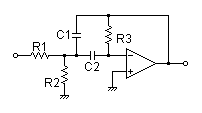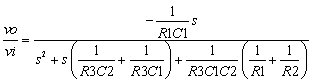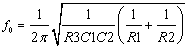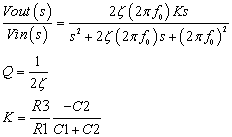# Multiple Feedback Band-pass Filter Design Tool

This page is a web application that design a multiple feedback band-pass filter. Use this utility to simulate the Transfer Function for filters at a given frequency, damping ratio ζ, Q or values of R and C. The response of the filter is displayed on graphs, showing Bode diagram, Nyquist diagram, Impulse response and Step response.

## Calculate the transfer function for multiple feedback band-pass filter with R and C values

 Vin(s)→→Vout(s)
Transfer function:R1=Ω C1=F R2=Ω C2=F R3=Ω
p:pico, n:nano, u:micro, k:kilo, M:mega

### Frequency analysis

Bode diagram
Phase  Group delay
Nyquist diagram
Pole, zero
Phase margin
Oscillation analysis

Upper and lower frequency limits:
f1= - f2=[Hz] (frequency limits are optional)

### Transient analysis

Step response
Impulse response
Overshoot
Final value of the step response

Simulation time:
0 - [sec] (optional)

## Calculate the R and C values for the multiple feedback filter at a given frequency and Q factor

 Vin(s)→→Vout(s)

Center frequency:Transfer function:K is gain at the center frequency.
Center frequency f0=Hz
Gain K= at f0. (K<0)

### Q factor | Damping ratio ζ

Quality factor Q =
Damping ratio ζ =
 C1 = F C2 = F
C1, C2 is optional. But when setting these capacitances, C1 and C2 of both are needed to give following the equation
(C2/C1) > 4ζ 2|K| - 1
(C2/C1) > |K|/Q2 - 1

Select Capacitor Sequence:
Select Resistor Sequence:

### Frequency analysis

Bode diagram
Phase  Group delay
Nyquist diagram
Pole, zero
Phase margin
Oscillation analysis

Upper and lower frequency limits:
f1= - f2=[Hz] (frequency limits are optional)

### Transient analysis

Step response
Impulse response
Overshoot
Final value of the step response

Simulation time:
0 - [sec] (optional)